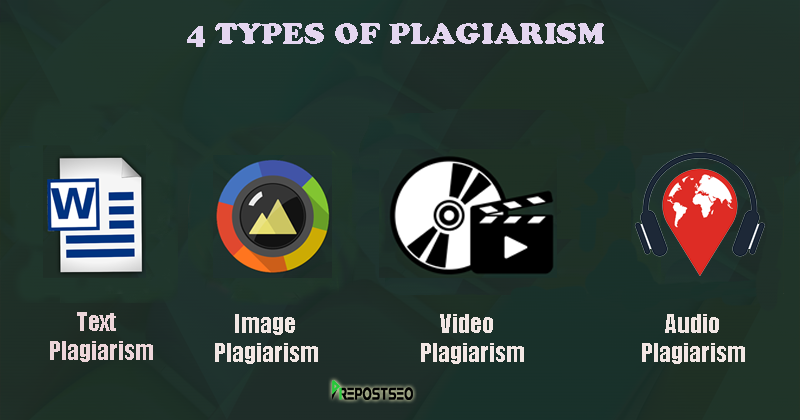There is also the option to spin capitalized words (assumed to be proper nouns) as well as leave any number of words unchanged, depending on whatever you enter into the "ignore" field, separated by commas. You also have the option to only keep the sentences that were altered a minimum percentage, as indicated by the "Keep Sentences that Changed" option.
!function(n,t){function r(e,n){return Object.prototype.hasOwnProperty.call(e,n)}function i(e){return void 0===e}if(n){var o={},s=n.TraceKit,a=[].slice,l="?";o.noConflict=function(){return n.TraceKit=s,o},o.wrap=function(e){function n(){try{return e.apply(this,arguments)}catch(e){throw o.report(e),e}}return n},o.report=function(){function e(e){l(),h.push(e)}function t(e){for(var n=h.length-1;n>=0;--n)h[n]===e&&h.splice(n,1)}function i(e,n){var t=null;if(!n||o.collectWindowErrors){for(var i in h)if(r(h,i))try{h[i].apply(null,[e].concat(a.call(arguments,2)))}catch(e){t=e}if(t)throw t}}function s(e,n,t,r,s){var a=null;if(w)o.computeStackTrace.augmentStackTraceWithInitialElement(w,n,t,e),u();else if(s)a=o.computeStackTrace(s),i(a,!0);else{var l={url:n,line:t,column:r};l.func=o.computeStackTrace.guessFunctionName(l.url,l.line),l.context=o.computeStackTrace.gatherContext(l.url,l.line),a={mode:"onerror",message:e,stack:[l]},i(a,!0)}return!!f&&f.apply(this,arguments)}function l(){!0!==d&&(f=n.onerror,n.onerror=s,d=!0)}function u(){var e=w,n=p;p=null,w=null,m=null,i.apply(null,[e,!1].concat(n))}function c(e){if(w){if(m===e)return;u()}var t=o.computeStackTrace(e);throw w=t,m=e,p=a.call(arguments,1),n.setTimeout(function(){m===e&&u()},t.incomplete?2e3:0),e}var f,d,h=[],p=null,m=null,w=null;return c.subscribe=e,c.unsubscribe=t,c}(),o.computeStackTrace=function(){function e(e){if(!o.remoteFetching)return"";try{var t=function(){try{return new n.XMLHttpRequest}catch(e){return new n.ActiveXObject("Microsoft.XMLHTTP")}},r=t();return r.open("GET",e,!1),r.send(""),r.responseText}catch(e){return""}}function t(t){if("string"!=typeof t)return[];if(!r(j,t)){var i="",o="";try{o=n.document.domain}catch(e){}var s=/(.*)\:\/\/([^:\/]+)([:\d]*)\/{0,1}([\s\S]*)/.exec(t);s&&s===o&&(i=e(t)),j[t]=i?i.split("\n"):[]}return j[t]}function s(e,n){var r,o=/function ([^(]*)\(([^)]*)\)/,s=/['"]?([0-9A-Za-z\$_]+)['"]?\s*[:=]\s*(function|eval|new Function)/,a="",u=10,c=t(e);if(!c.length)return l;for(var f=0;f0?s:null}function u(e){return e.replace(/[\-\[\]{}()*+?.,\\\^\$|#]/g,"\\\$&")}function c(e){return u(e).replace("<","(?:<|<)").replace(">","(?:>|>)").replace("&","(?:&|&)").replace('"','(?:"|")').replace(/\s+/g,"\\s+")}function f(e,n){for(var r,i,o=0,s=n.length;or&&(i=s.exec(o[r]))?i.index:null}function h(e){if(!i(n&&n.document)){for(var t,r,o,s,a=[n.location.href],l=n.document.getElementsByTagName("script"),d=""+e,h=/^function(?:\s+([\w\$]+))?\s*\(([\w\s,]*)\)\s*\{\s*(\S[\s\S]*\S)\s*\}\s*\$/,p=/^function on([\w\$]+)\s*\(event\)\s*\{\s*(\S[\s\S]*\S)\s*\}\s*\$/,m=0;m]+)>|([^\)]+))\((.*)\))? in (.*):\s*\$/i,o=n.split("\n"),l=[],u=0;u=0&&(g.line=v+x.substring(0,j).split("\n").length)}}}else if(o=d.exec(i[y])){var _=n.location.href.replace(/#.*\$/,""),T=new RegExp(c(i[y+1])),E=f(T,[_]);g={url:_,func:"",args:[],line:E?E.line:o,column:null}}if(g){g.func||(g.func=s(g.url,g.line));var k=a(g.url,g.line),A=k?k[Math.floor(k.length/2)]:null;k&&A.replace(/^\s*/,"")===i[y+1].replace(/^\s*/,"")?g.context=k:g.context=[i[y+1]],h.push(g)}}return h.length?{mode:"multiline",name:e.name,message:i,stack:h}:null}function y(e,n,t,r){var i={url:n,line:t};if(i.url&&i.line){e.incomplete=!1,i.func||(i.func=s(i.url,i.line)),i.context||(i.context=a(i.url,i.line));var o=/ '([^']+)' /.exec(r);if(o&&(i.column=d(o,i.url,i.line)),e.stack.length>0&&e.stack.url===i.url){if(e.stack.line===i.line)return!1;if(!e.stack.line&&e.stack.func===i.func)return e.stack.line=i.line,e.stack.context=i.context,!1}return e.stack.unshift(i),e.partial=!0,!0}return e.incomplete=!0,!1}function g(e,n){for(var t,r,i,a=/function\s+([_\$a-zA-Z\xA0-\uFFFF][_\$a-zA-Z0-9\xA0-\uFFFF]*)?\s*\(/i,u=[],c={},f=!1,p=g.caller;p&&!f;p=p.caller)if(p!==v&&p!==o.report){if(r={url:null,func:l,args:[],line:null,column:null},p.name?r.func=p.name:(t=a.exec(p.toString()))&&(r.func=t),"undefined"==typeof r.func)try{r.func=t.input.substring(0,t.input.indexOf("{"))}catch(e){}if(i=h(p)){r.url=i.url,r.line=i.line,r.func===l&&(r.func=s(r.url,r.line));var m=/ '([^']+)' /.exec(e.message||e.description);m&&(r.column=d(m,i.url,i.line))}c[""+p]?f=!0:c[""+p]=!0,u.push(r)}n&&u.splice(0,n);var w={mode:"callers",name:e.name,message:e.message,stack:u};return y(w,e.sourceURL||e.fileName,e.line||e.lineNumber,e.message||e.description),w}function v(e,n){var t=null;n=null==n?0:+n;try{if(t=m(e))return t}catch(e){if(x)throw e}try{if(t=p(e))return t}catch(e){if(x)throw e}try{if(t=w(e))return t}catch(e){if(x)throw e}try{if(t=g(e,n+1))return t}catch(e){if(x)throw e}return{mode:"failed"}}function b(e){e=1+(null==e?0:+e);try{throw new Error}catch(n){return v(n,e+1)}}var x=!1,j={};return v.augmentStackTraceWithInitialElement=y,v.guessFunctionName=s,v.gatherContext=a,v.ofCaller=b,v.getSource=t,v}(),o.extendToAsynchronousCallbacks=function(){var e=function(e){var t=n[e];n[e]=function(){var e=a.call(arguments),n=e;return"function"==typeof n&&(e=o.wrap(n)),t.apply?t.apply(this,e):t(e,e)}};e("setTimeout"),e("setInterval")},o.remoteFetching||(o.remoteFetching=!0),o.collectWindowErrors||(o.collectWindowErrors=!0),(!o.linesOfContext||o.linesOfContext<1)&&(o.linesOfContext=11),void 0!==e&&e.exports&&n.module!==e?e.exports=o:"function"==typeof define&&define.amd?define("TraceKit",[],o):n.TraceKit=o}}("undefined"!=typeof window?window:global)},"./webpack-loaders/expose-loader/index.js?require!./shared/require-global.js":function(e,n,t){(function(n){e.exports=n.require=t("./shared/require-global.js")}).call(n,t("../../../lib/node_modules/webpack/buildin/global.js"))}});
```SEOToolsCentre is a reliable name in the SEO services provider due to its high-quality SEO Tools. Paraphrase tool is our best product which works as a human writer. If you are got tired to write articles or to hire professional writers then you are at right place. It works as your classmate to create unique research papers, assignments and thesis for the educational purpose.
```

Country of origin for 26.1% of all visits is India. It lies approximately 8270 miles away from the server location (United States) and such a long distance can negatively affect website speed, as it takes some time for data to travel back and forth between those places. That’s why one of the best ways to speed up Spinnerchief.com page load time for the majority of users is moving the server to India or just closer to the user base.

After rewriting and keeping the dynamics of the writing same, our tool passes it through a plagiarism checker. This ensures that the legal standard of plagiarism is maintained after rewriting the article. After all this process, a final rewritten form is presented to you. All this process may require some time depending upon the nature and length of the article to be rewritten.
No! It is not necessary to use rewriting tool. It is designed for the people with poor English to fulfill their needs. If you are a professional content writer, then it’s also useful for you. It will enable you to increase your vocabulary and synonyms memory. Recently, we have added up to 500,000 synonyms in this tool for english language and it will enable you to create content with more professionally.

You have seen this type of article before - e.g. "10 Tips To Lose Weight" The key factor about this type of article is that each tip (each paragraph) makes sense in its own right - independently of the rest of the article. So if you can get a bunch of these paragraphs together, then you can mix any number of paragraphs in order to make your new article. The SpinnerChief TIPS plugin will do this task effortlessly for you. Check the details by clicking here

### Spin Rewriter 5.0 technology was a previous edition that allowed their clients to use the basic article rewriting system.  Their newer versions over the years have just kept getting better and better.   The new Spin Rewriter 9.0 technology gives you the option of assigning variables to your content.  Their software is dead easy to use.  In just three steps you can have up to 50 uniquely spun articles.

For this purpose, humans have created numerous rewriting tools to save time and use all words available in the English dictionary. Rewriting tools use millions of synonyms made available to them through artificial intelligence and instructions given by the human mind. If you hire the services of a content writer to rewrite/create content for you, they will charge you a lot. Big organizations can afford this but small startups and students can never afford the services of professional writers.#### ArticleRewriterTool.org is a free, programmed article rewriter that can efficiently rewrite human-readable text into a new and different version of a readable text. Content is the King; you must have heard; it is at least as important as SEO. An uninterrupted flow of fresh and human-readable content is the key to success for your blog or website as it helps to achieve search engine optimization. The use of article spinner or paraphrasing tool is not just limited to search engine optimization; you can use it to serve any purpose that requires the creation of fresh and unique content.

This is absolutely useless. It claims it rewrites English sentences, but it just offers words alternatives, which is only nouns. Also, most of those suggested alternatives are unable to use. If you see their maker's home page, Whitehat Box, you can see numerous people request the refund. It's far from western progressive software like Grammarly or sketch engine. They stole my money with the diceiptive advertisement.